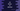# C# program to convert a binary value to decimal## C# program to convert a binary value to decimal:

Learn different ways to convert a binary value to decimal in C#. The binary number system uses base 2 to represent a value. It uses 1 and 0 to represent a number. Similarly, the decimal number system uses base 10. It uses 0, 1, 2, 3, 4, 5, 6, 7, and 9 to represent a number.

We can write our own algorithm or we can use the Convert.ToInt32 method to convert a binary value to decimal. In this post, we will learn both of these ways.

Before start writing the program, let’s understand how the algorithm works.

### Algorithm to convert a binary value to decimal:

We can use the below algorithm to convert a binary value to decimal:

• Initialize a variable as 0 to hold the final decimal value.
• Start from the rightmost digit of the binary value. Multiply it with 2^0 and add it to the decimal value.
• Move to the next digit to its left. Multiply it with 2^1 and add it to the decimal value. Similarly, iterate over all digits of the binary number.
• The calculated value is the required decimal value.

Let’s use this algorithm to convert 1011 to decimal.

• Rightmost digit is 1. 1 * 2^0 = 1. Sum = 1
• Next digit is 1. 1 * 2^1 = 2. Sum = 1 + 2 = 3
• Next digit is 0. 0 * 2^2 = 0. Sum = 3 + 0 = 3
• Next digit is 1. 1 * 2^3 = 8. Sum = 3 + 8 = 11

So, the final decimal value is 11.

We will use this algorithm to write the program.

### Method 1: Convert binary to decimal in C#:

This method will use the above algorithm to convert a binary value to decimal. It will take the binary value as an input from the user, convert it to decimal and print it out.

``````using System;

namespace dotnetsample
{
class Program
{
static int BinaryToDecimal(int binary)
{
int d = 0;
int remainder;
int multiplier = 1;

while (binary > 0)
{
remainder = binary % 10;
binary = binary / 10;
d += remainder * multiplier;
multiplier *= 2;
}
return d;
}
static void Main(string[] args)
{
int binary;

Console.WriteLine("Enter the binary value: ");

Console.WriteLine("Decimal Value: " + BinaryToDecimal(binary));

}
}
}``````

Here,

• BinaryToDecimal method is used to convert a binary value to decimal.
• We are reading the binary value as integer and passing it to this method. This method converts it to the decimal value and returns it.
• The while loop is used to calculate the decimal value. It finds the last digit of the number and multiplies it with the multiplier. It adds that value to d and removes the last digit of binary digit.
• d holds the final decimal value.

If you run this program, it will print outputs as like below:

``````Enter the binary value:
10101
Decimal Value: 21

Enter the binary value:
1011
Decimal Value: 11``````

### Method 2: Recursive way to convert a binary to decimal:

We can write a recursive method to convert a binary value to decimal. Let’s change the above program to use a recursive method:

``````using System;

namespace dotnetsample
{
class Program
{
static int BinaryToDecimal(int binary, int result, int multiplier)
{

if (binary == 0) return result;

int remainder = binary % 10;
result += remainder * multiplier;

return BinaryToDecimal(binary / 10, result, multiplier * 2);
}
static void Main(string[] args)
{
int binary;

Console.WriteLine("Enter the binary value: ");

Console.WriteLine("Decimal Value: " + BinaryToDecimal(binary, 0, 1));

}
}
}``````
• BinaryToDecimal method is changed to a recursive method.
• It takes the binary value, result and multiplier as the parameters. If binary become 0, it returns result.
• On each recursive call, we are removing the last digit of binary, updating the value of result and multiplying the multiplier value by 2.

It will give similar output.

### Method 3: By using Convert.ToInt32 method:

The ToInt32 method can be used to convert a binary number to decimal. It accepts two parameters. The first one is the string value of the binary number and the second one is the base for the conversion, i.e. 2.

``````using System;

namespace dotnetsample
{
class Program
{
static void Main(string[] args)
{
int binary;

Console.WriteLine("Enter the binary value: ");